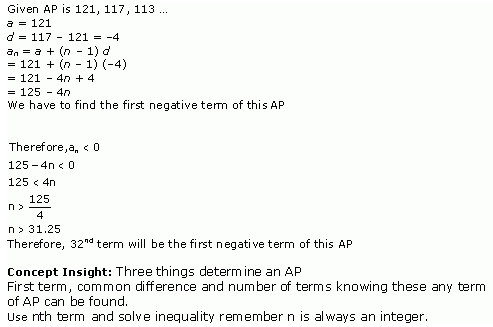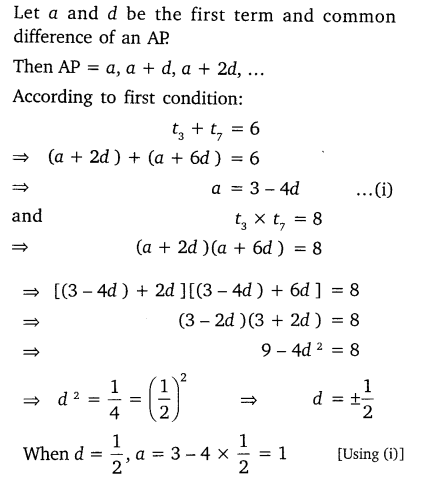# NCERT Solutions for Class 10 Maths Chapter 5 Arithmetic Progressions Ex 5.4

Get Free NCERT Solutions for Class 10 Maths Chapter 5 Ex 5.4 PDF. Arithmetic Progressions Class 10 Maths NCERT Solutions are extremely helpful while doing your homework. Exercise 5.4 Class 10 Maths NCERT Solutions were prepared by Experienced ncert-books.in Teachers. Detailed answers of all the questions in Chapter 5 Maths Class 10 Arithmetic Progressions Exercise 5.4 provided in NCERT TextBook.

Topics and Sub Topics in Class 10 Maths Chapter 5 Arithmetic Progressions:

 Section Name Topic Name 5 Arithmetic Progressions 5.1 Introduction 5.2 Arithmetic Progressions 5.3 Nth Term Of An AP 5.4 Sum Of First N Terms Of An AP 5.5 Summary

You can also download the free PDF of  Ex 5.4 Class 10 Arithmetic Progressions NCERT Solutions or save the solution images and take the print out to keep it handy for your exam preparation.

 Board CBSE Textbook NCERT Class Class 10 Subject Maths Chapter 5 Chapter Name Arithmetic Progressions Exercise Ex 5.4 Number of Questions Solved 5 Category NCERT Solutions

## NCERT Solutions for Class 10 Maths Chapter 5 Arithmetic Progressions Ex 5.4

NCERT Solutions for Class 10 Maths Chapter 5 Arithmetic Progressions Ex 5.4 are part of NCERT Solutions for Class 10 Maths. Here we have given NCERT Solutions for Class 10 Maths Chapter 5 Arithmetic Progressions Exercise 5.4

Question 1.
Which term of the AP: 121, 117. 113, ….., is its first negative term?
Solution:Question 2.
The sum of the third term and the seventh term of an AP is 6 and their product is 8. Find the sum of first sixteen terms of the AP?
Solution:Download NCERT Solutions For Class 10 Maths Chapter 5 Arithmetic Progressions PDF

Question 3.
A ladder has rungs 25 cm apart. The rungs decrease uniformly in length from 45 cm at the bottom to 25 at the top. If the top and the bottom rungs are${ 2 }\frac { 1 }{ 2 } m$apart, what is the length of the wood required for the rungs?Solution:Question 4.
The houses of a row are numbered consecutively from 1 to 49. Show that there is value of x such that the sum of the numbers of the houses preceding the house numbered x is equal to the sum of the numbers of the houses following it. Find this value of x.
Solution:Question 5.
A small terrace at a football ground comprises of 15 steps each of which is 50  m long and built of solid concrete. Each step has a rise of$\frac { 1 }{ 2 } m$and a tread of$\frac { 1 }{ 2 } m$. Calculate the total volume of concrete required to build the terrace.Solution:error: Content is protected !!
+